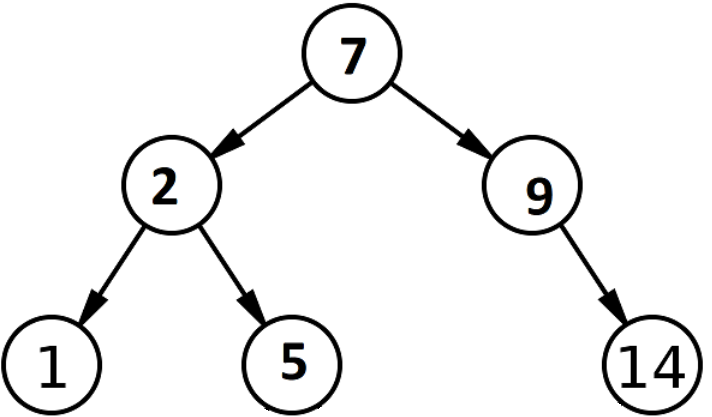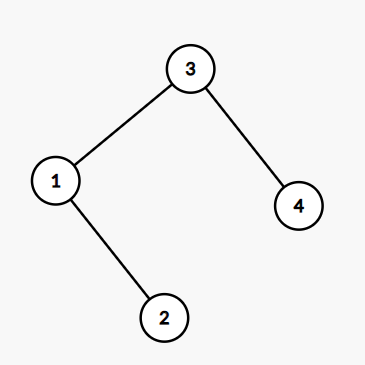Problem title
Difficulty
Avg time to solve

Homogenous Substrings
Easy
15 mins
Moderate
30 mins
Greatest Binary String
Easy
15 mins
One Segment
Easy
10 mins
Stream Of Characters
Hard
50 mins
Implement Map Sum Pair
Moderate
30 mins
Maximize the average working ratio
Moderate
15 mins
Car Pooling
Moderate
30 mins
Create Lexicographically Greatest Array
Hard
45 mins
Ninja And Divisible Array
Moderate
15 mins

# Counts the Nodes

Difficulty: EASY
Avg. time to solve
25 min
Success Rate
70%

Problem Statement

#### A BST is defined as follows:

``````The left subtree of a node contains only nodes with keys less than or equal to the node's key.
The right subtree of a node contains only nodes with keys greater than or equal to the node's key.
Both the left and right subtrees must also be binary search trees.

Example of a BST
``````##### Input Format-
``````First-line contains ‘T’, denoting the number of Test cases.
For each Test case:
The first line contains an integer ‘N’, denoting the number of nodes in the BST.
The following line will contain the values of the tree’s nodes in the level order form ( -1 for 'NULL' node). Refer to the example for further clarification.
The following line contains two space-separated integers, ‘L’ and ‘R’.
``````

#### The input of the tree depicted in the image above will be like :

``````6 → represents the total number of nodes.
7 2 9 1 5 -1 14 -1 -1 -1 -1 -1 -1 → represents the level order of Tree.
Explanation :
Level 1 :
The root node of the tree is 7.

Level 2 :
The left child of 7 = 2.
The right child of 7 = 9.

Level 3 :
The left child of 2 = 1.
The right child of 7 = 5.
The left child of 9 = null (-1).
The right child of 9 = 14.

Level 4:
The left child of 1 = null (-1).
The right child of 1 = null (-1).
The left child of 5 = null (-1).
The right child of 5 = null (-1).
The left child of 14 = null (-1).
The right child of 14 = null (-1).
``````
##### Output Format-
``````For each test case, print an integer denoting the number of rooms you raided.
``````
##### Note :
``````You don’t need to print anything. It has already been taken care of. Just implement the given function.
``````
##### Constraints -
``````1 <= ‘T’ <= 5
1 <= ‘N’ <= 10^5
1 <= ‘X’ <= 10^5, for 1 <= i <= ‘N’
1 <= ‘L’ <= ‘R’ <= 10^5
Note- the sum of ‘N’ over all test cases does not exceed 10^5.

Time Limit: 1 sec
``````
##### Sample Input-1
``````2
6
7 2 9 1 5 -1 14 -1 -1 -1 -1 -1 -1
5 9
4
3 1 4 -1 2 -1 -1 -1 -1
3 6
``````
##### Sample Output-1
``````3
2
``````
##### Explanation for Sample Input 1:
``````For test case 1:
The Input is for the given image. From the image, we get nodes [7, 9, 5] that satisfy the condition. Hence the answer is 3.

For test case 2:
````````````From the image, we get nodes [3, 4] that satisfy the condition hence that the answer is 2.
``````
##### Sample Input -2
``````2
3
6 4 14 -1 -1 -1 -1
10 14
3
12 11 13 -1 -1 -1 -1
1 10
``````
##### Sample Output -2
``````1
0
``````Console